# Thermistor

### From DT Online

##### Description

The resistance of a Thermistor decreases when they are heated (negative temperature coefficient type).

##### Features and Applications

Typical resistance values are 1 kilohm at 25°C and 80 ohms at 100°C.

Thermistors are suitable for temperature controlled switches and temperature measurement.

A signal of 0.6V, or greater, to the BASE of the transistor "switches on" the transistor and allows a current to flow in the COLLECTOR / EMITTER circuit.Transistors also act as CURRENT AMPLIFIERS since a small base current (Ib) controls a much larger collector current (Ic). The amplification (GAIN - hfe) of a transistor, is given by the equation hfe = Ic/Ib and is typically several hundreds. (Note that the use of a variable resistance in the other half of the potential divider circuit allows the sensitivity of the circuit to be adjusted).

##### Heat Sensor

Many "control" circuits are triggered by a signal which is produced by a "potential divider" network. The signal value depends upon the component values and the supply voltage:

Vs=V*R2/(R1+R2)

where:

• Vs is the signal value
• V is the supply voltage
• R1 is the resistance between the positive supply rail and the base of the transistor
• R2 is the resistance between the negative supply rail and the base of the transistor

The resistance of a thermistor decreases when it is heated. As heat increases, therefore, the resistance of the thermistor decreases and allows the voltage at the base of the transistor to move closer to that of the positive supply rail.

##### Cold Sensor

As with the Heat Sensor, this circuit is triggered by a signal which is produced by a "potential divider" network and the signal value depends upon the component values and the supply voltage:

Vs=V*R2/(R1+R2)

where:

• Vs is the signal value
• V is the supply voltage
• R1 is the resistance between the positive supply rail and the base of the transistor
• R2 is the resistance between the negative supply rail and the base of the transistor

The resistance of a thermistor decreases when it is heated. In this position therefore, as heat decreases the resistance of the thermistor increases and allows the voltage at the base of the transistor to move closer to that of the positive supply rail.

Note:

• When choosing a transistor it is important to make sure that the gain is high enough for the required use and that the maximum current which it can handle is sufficient to drive the output device. e.g. BFY 51 gain > 40 , max Ic = 1 amp.
• If the max Ic value is exceeded, the transistor will probably "burn out".
• The transistor acts as a NOT gate (inverter) since a "high" at the base gives a "low" at the collector. (i.e. when the transistor switches on, the collector voltage decreases).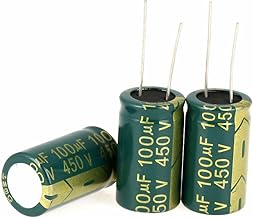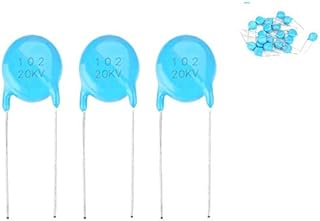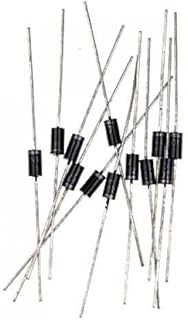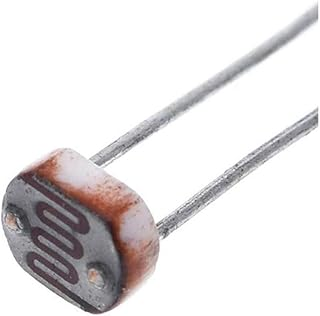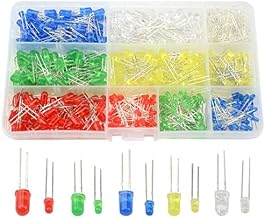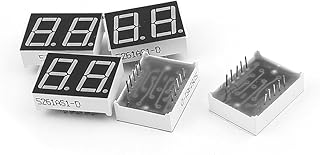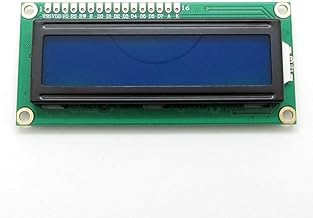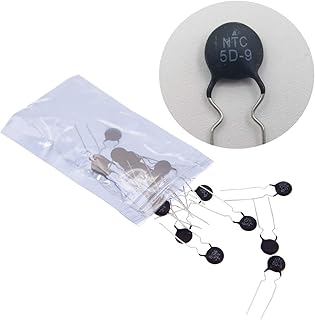1/4w Resistors PackElectrolytic CapacitorsCeramic Disc CapacitorsRectifier DiodesPhoto Light Sensitive Resistor (LDR)Light Emitting Diodes (LED)Common Cathode LED DisplayLCD Module DisplayThermistor Resistor